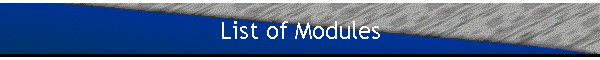• #### Review: The Fundamental Assumptions of Quantum Mechanics

• A free particle, one-dimensional wave packets, the Heisenberg uncertainty relations, the time evolution of a free wave packet, the spreading of a wave packet
• #### Review: Square Potentials

• A particle in a time-independent scalar potential, potential steps, square wells, d-function potentials
• #### The WKB Approximation

• The WKB approximations for bound states
• #### Mathematical Foundations of Quantum Mechanics

• Linear vector spaces, Dirac notation, subspaces, linear operators, Hermitian operators, unitary operators, function of operators, representations in state space, change of representation, the eigenvalue problem, commuting observables, the |r> and |p> representations
• #### The Postulates of Quantum Mechanics

• Postulates, mean value and root-mean-square deviation, conservation of probability, the evolution operator
• #### Representations

• The Schroedinger picture, the Heisenberg picture, the interaction picture, time-dependent perturbation theory, the evolution of the mean value of an observable, interference effects
• #### The Density Matrix

• A statistical mixture of states, the density operator, the physical meaning of the density matrix and the density operator
• #### The Harmonic Oscillator

• The eigenvalues and eigenfunctions of the 1D harmonic oscillator, the mean value and root mean square deviation of X and P, coherent states, tensor product spaces, the 3D harmonic oscillator
• #### Two-Level Systems (A Spin 1/2 particle)

• Spin, a spin 1/2 particle in a uniform magnetic field, a general study of a two-level system
• #### Two Spin 1/2 particles

• The tensor product space of two spin 1/2 particles
• #### Angular Momentum

• Commutation Relations, basis states, orbital angular momentum, the spherical harmonics
• #### Symmetries and Constants of Motion

• The translation operator, the rotation operator, rotation of observables, scalar and vector observables, spinors, the rotation operator for a spin 1/2 particle